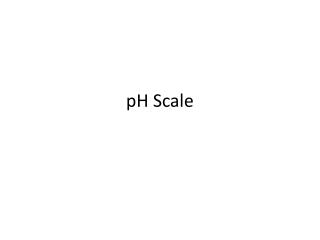# pH Scale - PowerPoint PPT PresentationDownload PresentationpH Scale

pH ScaleDownload Presentation## pH Scale

- - - - - - - - - - - - - - - - - - - - - - - - - - - E N D - - - - - - - - - - - - - - - - - - - - - - - - - - -
##### Presentation Transcript

1. pH Scale

2. Ion product constant for water Kw H2O  H+ + OH- Kw = [H+] [OH-] = 1.0 X 10-14 mol/L Since [H+] = [OH-] for water Then each equals 1.0 X 10-7 M Water is neutral (neither acid or base)

3. The [H+] or [OH-] can be calculated if one is known…. Example: if [H+] = 1.0 X 10-5 Then, [OH-] = 1.0 X 10-9 because [H+] [OH-] must = 1.0 X 10-14

4. If the [H+] is larger than the [OH-] then the solution is acid If the [OH-] is larger than the [H+], then the solution is basic

5. pH Concept that describes how acidic or how basic a solution is… pH = -log [H+] Defined in terms of H+ pH < 7 acid pH > 7 base

6. pH scale acidic basic 0 7 14 [H+] = [OH-] 10-7 = 10-7 [H+] > 1.0 X 10-7 [OH-] > 1.0 X 10-7

7. http://www.quia.com/rd/1975.html

8. pOH pOH = -log [OH-] pH + pOH = 14 14 – pOH = pH